Courses

# Equations and Matrices (Part - 3) CA CPT Notes | EduRev

## Business Mathematics and Logical Reasoning & Statistics

Created by: Sushil Kumar

## CA CPT : Equations and Matrices (Part - 3) CA CPT Notes | EduRev

The document Equations and Matrices (Part - 3) CA CPT Notes | EduRev is a part of the CA CPT Course Business Mathematics and Logical Reasoning & Statistics.
All you need of CA CPT at this link: CA CPT

SUMMARY

In this unit basic applications to matrices and determinates has been studied. Matrix is

defined. Some special types of matrices are mentioned. Operations of matrices dealt with.

Determinants are defined and their properties are discussed. The methods Cramer’s rule.

1) General form matrix of order m × n is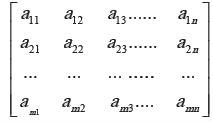2) Only square matrices have determinates. A Determinant of n rows and n columns is

called determinant of order n . General form of determinant of order n is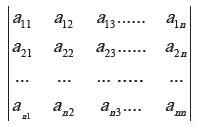3) Only matrices of the same order can be added or subtracted. To add (or subtract) two

matrices, we add (or subtract) their corresponding elements.

4) To multiply a matrix with a number, we multiply every element of the matrix with that

number whereas to multiply a determinant with a number we multiply only one row (or

column) of the determinant with that number.

5) Two matrices can be multiplied only if the number of columns of the first is the same as

the number of rows of the second, E.g. , a 2 × 3 matrix can be multiplied by a 3 × 4 matrix.

The order of resulting matrix will be 3 × 4.

6) Transpose of a matrix A is the matrix obtained by interchanging rows and columns of the matrix A. It is denoted by A’or AT .

7) Adjoint of matrix A is transpose of the co-factor matrix of A, e.g.,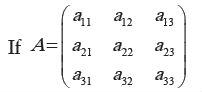Let C11 be co-factor of a11 and so on
Then Co-factor matrix of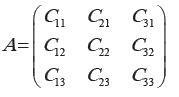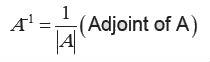69 docs|76 tests

,

,

,

,

,

,

,

,

,

,

,

,

,

,

,

,

,

,

,

,

,

;